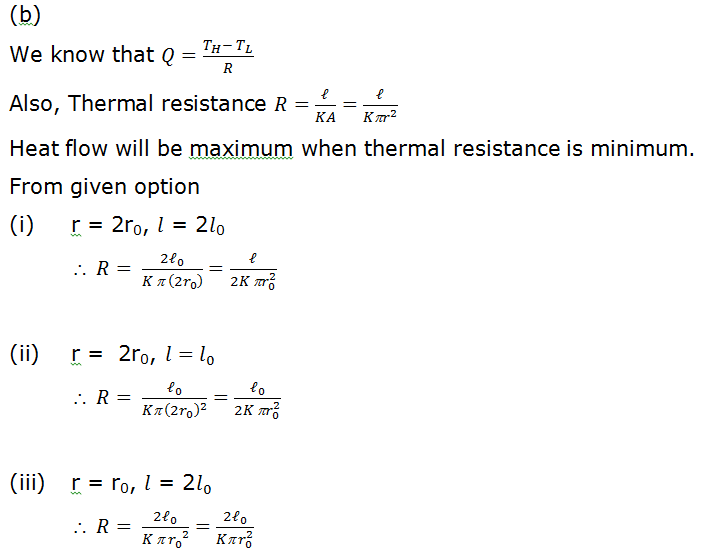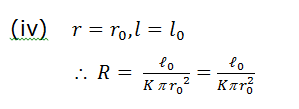# Which of the following circular rods (given radius r and length l), each made of the same materia and whose ends are maintained at the same temperature will conduct most heat?     (a)     r = r0; l=l0     (b)    r = 2r0; l=l_0      (c)    r = r0; l=2l_0

8 years agoIt is clear that for option (2) resistance is minimum, hence, heat flow will be maximum

[Alt :

(i)     Rate of heat flow is directly proportiona to area

(ii)    Inversely proportional to length.

:. Heat flow will be maximum when r is maximum and l is minimum.